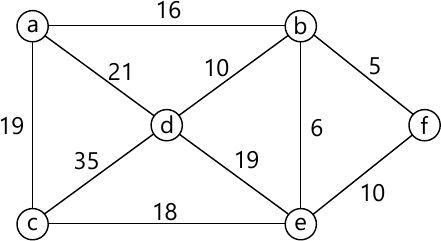MORE IN Data Structure & Algorithm Analysis
MU Information Technology (Semester 3)
Data Structure & Algorithm Analysis
December 2015
Total marks: --
Total time: --
INSTRUCTIONS
(1) Assume appropriate data and state your reasons
(2) Marks are given to the right of every question
(3) Draw neat diagrams wherever necessary

1 (a) Explain with example
i) Degree of tree
ii) Height of tree
iii) Depth of tree
3 M
1 (b) What is linked list? Give its applications.
3 M
1 (c) Define Graph. List the types Graph with example.
3 M
1 (d) What is Asymptotic Notations.
3 M
1 (e) Write down the properties of Red-Black trees.
3 M
1 (f) What are linear and non-linear data structures.
3 M
1 (g) Define minimum spanning tree. List the techniques to compute minimum spanking tree.
2 M

2 (a) Write a program to implement Queue ADT using array.
10 M
2 (b) Define Binary search tree. Write an algorithm to implement Insertion and Deletion Operation.
10 M

3 (a) Write a program to convert INFIX expression into POSTFIX expression.
10 M
3 (b) Define AVL tree? Construct AVL tree for following data [ Mention type of rotation for each case]
1, 2, 3, 4, 8, 7, 6, 5, 11, 10, 12.
10 M

4 (a) Using Prim's and Kruskal's algorithm find minimum spanning tree for the following Graph.10 M
4 (b) Write an algorithm to implement shell sort.
10 M

5 (a) Write a program to create singly linked list and display the list.
10 M
5 (b) Explain BFS and DFS algorithm with example.
10 M

Write short note on any four.
6 (a) B-Tree
5 M
6 (b) Red Black Trees
5 M
6 (c) Searching Algorithms
5 M
6 (d) Sparse Matrix
5 M
6 (e) Euclid's algorithm
5 M
6 (f) Marge Sort
5 M

More question papers from Data Structure & Algorithm Analysis